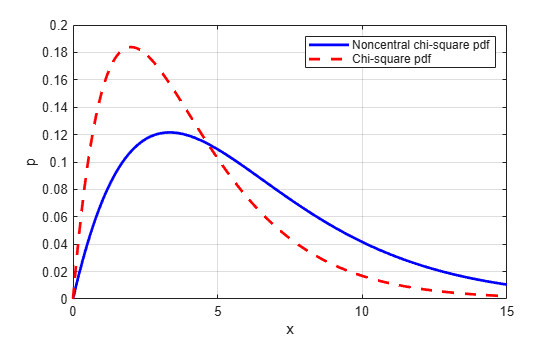## Noncentral Chi-Square Distribution

### Definition

There are many equivalent formulas for the noncentral chi-square distribution function. One formulation uses a modified Bessel function of the first kind. Another uses the generalized Laguerre polynomials. The cumulative distribution function is computed using a weighted sum of χ2 probabilities with the weights equal to the probabilities of a Poisson distribution. The Poisson parameter is one-half of the noncentrality parameter of the noncentral chi-square

`$F\left(x|\nu ,\delta \right)=\sum _{j=0}^{\infty }\left(\frac{{\left(\frac{1}{2}\delta \right)}^{j}}{j!}{e}^{\frac{-\delta }{2}}\right)\mathrm{Pr}\left[{\chi }_{\nu +2j}^{2}\le x\right]$`

where δ is the noncentrality parameter.

### Background

The χ2 distribution is actually a simple special case of the noncentral chi-square distribution. One way to generate random numbers with a χ2 distribution (with ν degrees of freedom) is to sum the squares of ν standard normal random numbers (mean equal to zero.)

What if the normally distributed quantities have a mean other than zero? The sum of squares of these numbers yields the noncentral chi-square distribution. The noncentral chi-square distribution requires two parameters: the degrees of freedom and the noncentrality parameter. The noncentrality parameter is the sum of the squared means of the normally distributed quantities.

The noncentral chi-square has scientific application in thermodynamics and signal processing. The literature in these areas may refer to it as the Rician Distribution or generalized Rayleigh Distribution.

### Examples

#### Compute Noncentral Chi-Square Distribution pdf

Compute the pdf of a noncentral chi-square distribution with degrees of freedom `V = 4` and noncentrality parameter `DELTA = 2`. For comparison, also compute the pdf of a chi-square distribution with the same degrees of freedom.

```x = (0:0.1:10)'; ncx2 = ncx2pdf(x,4,2); chi2 = chi2pdf(x,4);```

Plot the pdf of the noncentral chi-square distribution on the same figure as the pdf of the chi-square distribution.

```figure; plot(x,ncx2,'b-','LineWidth',2) hold on plot(x,chi2,'g--','LineWidth',2) legend('ncx2','chi2')```